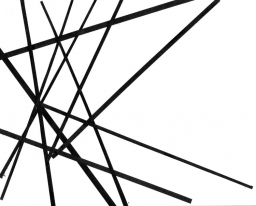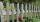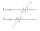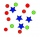# Divide in ratio

Line segment AB 12 cm long divide in a ratio of 5: 3. How long are the individual parts?

a =  7.5 cm
b =  4.5 cm

### Step-by-step explanation:Did you find an error or inaccuracy? Feel free to write us. Thank you!Tips to related online calculators
Need help to calculate sum, simplify or multiply fractions? Try our fraction calculator.
Check out our ratio calculator.
Do you want to convert length units?

## Related math problems and questions:

• DividingDivide the three-line segment 13 cm, 26 cm, and 19.5 cm long for parts so that the individual pieces were equally long and longest. How long will the individual parts, and how many will it?
• Line segmentLine segment AB is 8 cm long. Divide it in a ratio of 2: 3.
• Line segmentCut a line segment of 15 cm into two line segments so that their lengths are in ratio 2:1. What length will each have?
• Line segmentThe 4 cm long line segment is enlarged in the ratio of 5/2. How many centimeters will measure the new line segment?
• SegmentsLine segments 62 cm and 2.2 dm long we divide into equal parts which lengths in centimeters is expressed integer. How many ways can we divide?
• HexagonDivide a regular hexagon into lines into nine completely identical parts; none of them must be in a mirror image (individual parts can only be rotated arbitrarily).
• Points on line segmentPoints P & Q belong to segment AB. If AB=a, AP = 2PQ = 2QB, find the distance: between point A and the midpoint of the segment QB.
• Map 2At what scale is made map if the distance 8.2 km corresponds on the map segment 5 cm long?
• 2d shapeCalculate the content of a shape in which an arbitrary point is not more than 3 cm from the segment AB. The length of the segment AB is 5 cm.
• MO Z8–I–6 2018In the KLMN trapeze, KL has a 40 cm base and an MN of 16 cm. Point P lies on the KL line so that the NP segment divides the trapezoid into two parts with the same area. Find the length of the KP line.
• DivideDivide the number 72 in the ratio 7: 2 and calculate the ratio of the numbers found in this order and write down as decimal.
• Divide an isosceles triangleHow to divide an isosceles triangle into two parts with equal contents perpendicular to the axis of symmetry (into a trapezoid and a triangle)?
• DivideDivide substance 110 cm long to two parts so that it first part is 10 cm longer than the second part and one part will be 10 times longer than the second portion. How long the parts will be?
• Scale of the mapDetermine the map's scale, which is the actual distance of 120 km l represented by a segment long 6 cm.
• Divide 5Divide 288 in the following ratio 3 : 4 : 5
• SegmentsWhich of the pairs of numbers on the number line encloses the longest segment: ?
• Sides od triangleSides of the triangle ABC has length 4 cm, 5 cm and 7 cm. Construct triangle A'B'C' that are similar to triangle ABC which has a circumference of 12 cm.